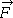(a) At a certain instant, a particle-like object is acted on by a force= (3.7N)(2.4 N)+ (9.3 N)while the object’s velocity is= −(2.0 m/s)+ (4.0 m/s). What is the instantaneous rate at which the force does work on the object?

(b) At some other time, the velocity consists of only a y component. If the force is unchanged and the instantaneous power is −12 W, what is the velocity of the object? (Express your answer in vector form.)